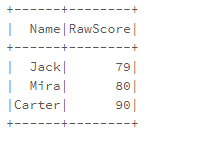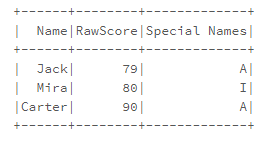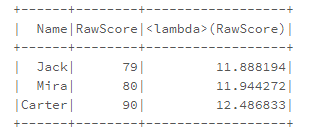# How to Write Spark UDF (User Defined Functions) in Python ?

• Last Updated : 06 Jun, 2021

In this article, we will talk about UDF(User Defined Functions) and how to write these in Python Spark. UDF, basically stands for User Defined Functions. The UDF will allow us to apply the functions directly in the dataframes and SQL databases in python, without making them registering individually. It can also help us to create new columns to our dataframe, by applying a function via UDF to the dataframe column(s), hence it will extend our functionality of dataframe. It can be created using the udf() method.

udf(): This method will use the lambda function to loop over data, and its argument will accept the lambda function, and the lambda value will become an argument for the function, we want to make as a UDF.

### Sample Pyspark Dataframe

Let’s create a dataframe, and the theme of this dataframe is going to be the name of the student, along with his/her raw scores in a test out of 100.

## Python3

 `from` `pyspark.sql ``import` `SparkSession ` `from` `pyspark.sql.types ``import` `IntegerType,StringType ` `from` `pyspark.sql.functions ``import` `udf ` ` `  `spark ``=` `SparkSession.builder.appName(``'UDF PRACTICE'``).getOrCreate() ` ` `  `cms ``=` `[``"Name"``,``"RawScore"``] ` `data ``=`  `[(``"Jack"``, ``"79"``), ` `        ``(``"Mira"``, ``"80"``), ` `        ``(``"Carter"``, ``"90"``)] ` `                    `  `df ``=` `spark.createDataFrame(data``=``data,schema``=``cms) ` ` `  `df.show()`

Output:### Creating Sample Function

Now, we have to make a function. So, for understanding, we will make a simple function that will split the columns and check, that if the traversing object in that column(is getting equal to ‘J'(Capital J) or ‘C'(Capital C) or ‘M'(Capital M), so it will be converting the second letter of that word, with its capital version. The implementation of this code is:

## Python3

 `def` `Converter(``str``): ` `    ``result ``=` `"" ` `    ``a ``=` `str``.split(``" "``) ` `     `  `    ``for` `q ``in` `a: ` `        ``if` `q ``=``=` `'J'` `or` `'C'` `or` `'M'``: ` `            ``result ``+``=` `q[``1``:``2``].upper() ` `             `  `    ``return` `result `

### Making UDF from Sample function

Now, we will convert it to our UDF function, which will, in turn, reduce our workload on data. For this, we are using lambda inside UDF.

## Python3

 `NumberUDF ``=` `udf(``lambda` `m: Converter(m))`

### Using UDF over Dataframe

The next thing we will use here, is the withcolumn(), remember that withcolumn() will return a full dataframe. So we will use our existing df dataframe only, and the returned value will be stored in df only(basically we will append it).

## Python3

 `df.withColumn(``"Special Names"``, NumberUDF(``"Name"``)).show() `

Output:Note: We can also do this all stuff in one step.

### UDF with annotations

Now, a short and smart way of doing this is to use “ANNOTATIONS”(or decorators). This will create our UDF function in less number of steps. For this, all we have to do use @ sign(decorator) in front of udf function, and give the return type of the function in its argument part,i.e assign returntype as Intergertype(), StringType(), etc.

## Python3

 `@udf``(returnType``=``StringType()) ` `def` `Converter(``str``): ` `    ``result ``=` `"" ` `    ``a ``=` `str``.split(``" "``) ` `     `  `    ``for` `q ``in` `a: ` `        ``if` `q ``=``=` `'J'` `or` `'C'` `or` `'M'``: ` `            ``result ``+``=` `q[``1``:``2``].upper() ` `        ``else``: ` `            ``result ``+``=` `q ` `    ``return` `result ` ` `  ` `  `df.withColumn(``"Special Names"``, Converter(``"Name"``)) \ ` `    ``.show() `

Output:Example:

Now, let’s suppose there is a marking scheme in the school that calibrates the marks of students in terms of its square root added 3(i.e they will be calibrating the marks out of 15). So, we will define a UDF function, and we will specify the return type this time. i.e float data type. So, declaration of this function will be–

## Python3

 `def` `SQRT(x): ` `    ``return` `float``(math.sqrt(x)``+``3``) `

Now, we will define an udf, whose return type will always be float,i.e we are forcing the function, as well as the UDF to give us result in terms of floating-point numbers only. The definition of this function will be –

## Python3

 `UDF_marks ``=` `udf(``lambda` `m: SQRT(m),FloatType()) `

The second parameter of udf,FloatType() will always force UDF function to return the result in floatingtype only. Now, we will use our udf function, UDF_marks on the RawScore column in our dataframe, and will produce a new column by the name of”<lambda>RawScore”, and this will be a default naming of this column. The code for this will look like –

## Python3

 `df.select(``"Name"``,``"RawScore"``, UDF_marks(``"RawScore"``)).show() `

Output:My Personal Notes arrow_drop_up
Recommended Articles
Page :